Machine learning pipeline
Machine learning pipeline tutorialIn this tutorial, we’ll create a pipeline that does the following:

1. Load data from an online endpoint
2. Select columns and fill in missing values
3. Train a model to predict which passengers will survive

If you prefer to skip the tutorial and view the finished code, follow this guide.

If you haven’t setup a project before, check out the setup guide before starting.

## ​1. Setup

### ​1a. Add Python packages to project

In the left sidebar (aka file browser), click on the `requirements.txt` file under the `demo_project/` folder.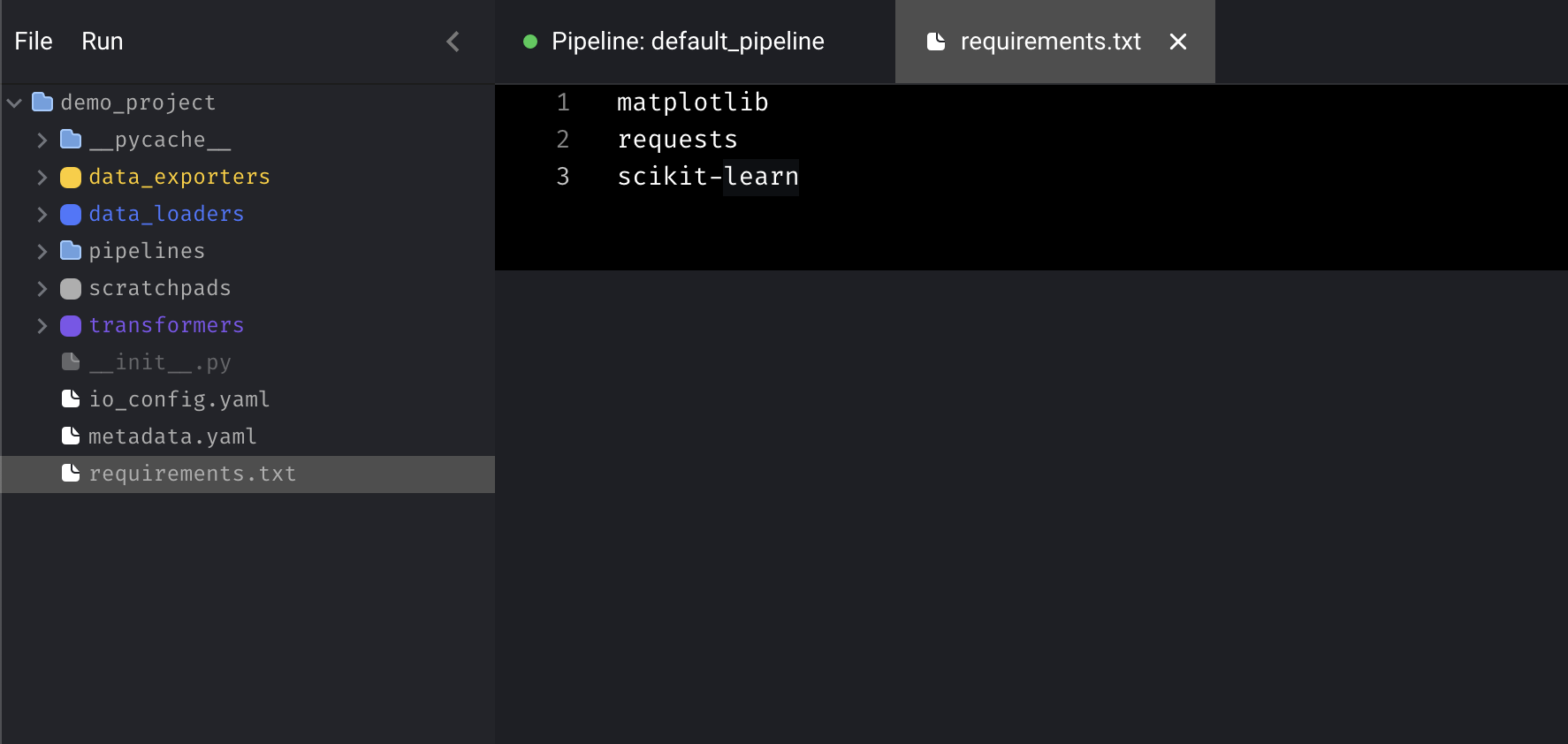Then add the following dependencies to that file:

``````matplotlib
requests
scikit-learn
``````

Then, save the file by pressing `⌘ + S`.

### ​2a. Install dependencies

The simplest way is to run pip install from the tool.

Add a scratchpad block by pressing the `+ Scratchpad` button. Then run the following command:

``````pip install -r demo_project/requirements.txt
``````

Alternatively, here are other ways of installing dependencies (depending on if you are using Docker or not):

#### ​Docker

Get the name of the container that is running the tool:

``````docker ps
``````

Sample output:

Sample output
``````CONTAINER ID   IMAGE       COMMAND                  CREATED         STATUS         PORTS     NAMES
214e1155f5c3   mage/data   "python mage_ai/comm…"   5 seconds ago   Up 2 seconds             mage-ai_server_run_6f8d367ac405
``````

The container name in the above sample output is `mage-ai_server_run_6f8d367ac405`.

Then run this command to install Python packages in the `demo_project/requirements.txt` file:

``````docker exec [container_name] pip3 install -r demo_project/requirements.txt
``````

### ​pip

If you aren’t using Docker, just run the following command in your terminal:

``````pip3 install -r demo_project/requirements.txt
``````

## ​2. Create new pipeline

In the top left corner, click `File > New pipeline`. Then, click the name of the pipeline next to the green dot to rename it to `titanic survivors`.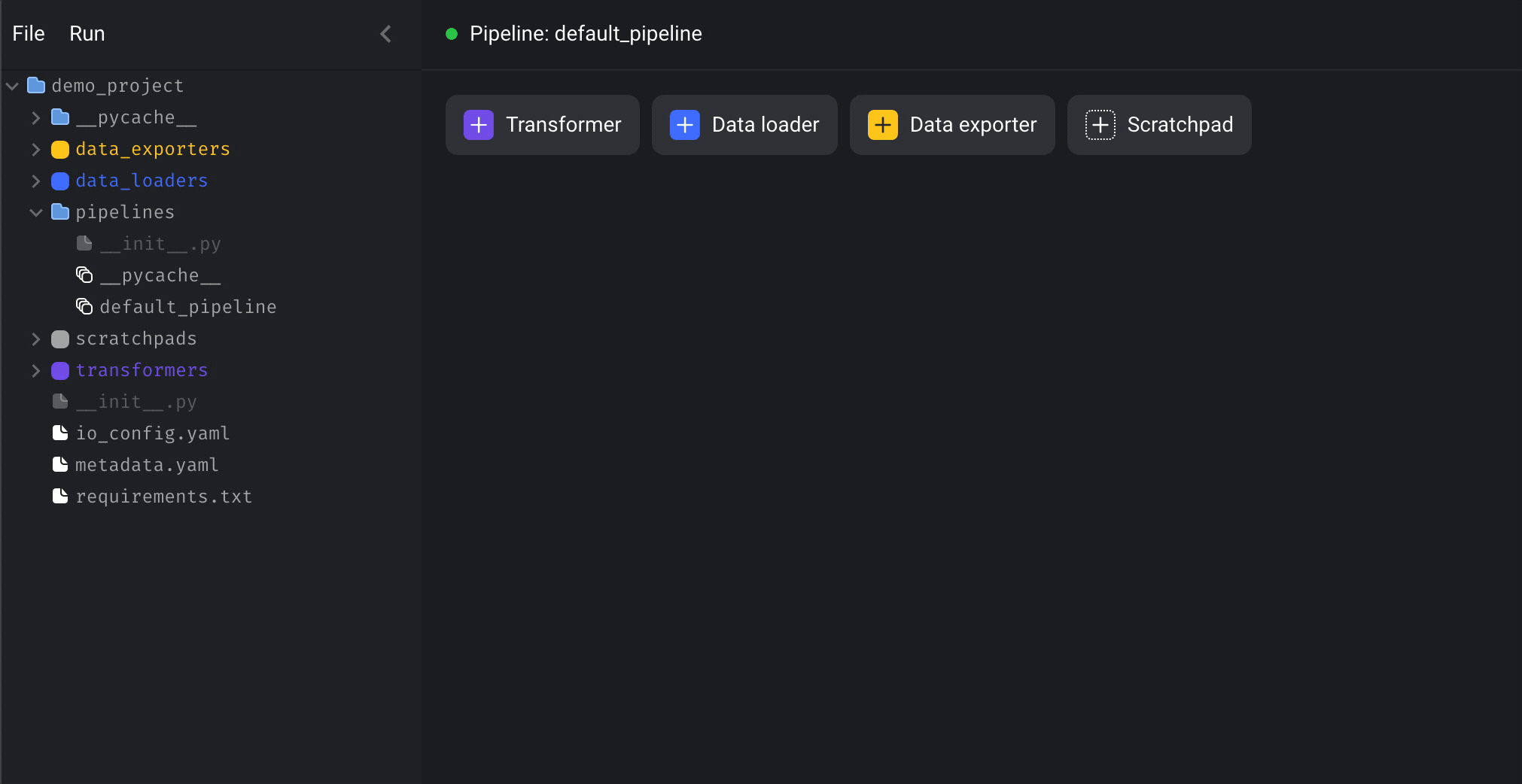## ​3. Play around with scratchpad

There are 4 buttons, click on the `+ Scratchpad` button to add a block.

Paste the following sample code in the block:

``````import matplotlib.pyplot as plt
import numpy as np

t = np.arange(0.0, 2.0, 0.01)
s = 1 + np.sin(2*np.pi*t)
plt.plot(t, s)

plt.xlabel('time (s)')
plt.ylabel('voltage (mV)')
plt.title('About as simple as it gets, folks')
plt.grid(True)
plt.show()
``````

Then click the `Play button` on the right side of the block to run the code. Alternatively, you can use the following keyboard shortcuts to execute code in the block:

• ⌘ + Enter
• Control + Enter
• Shift + Enter (run code and add a new block)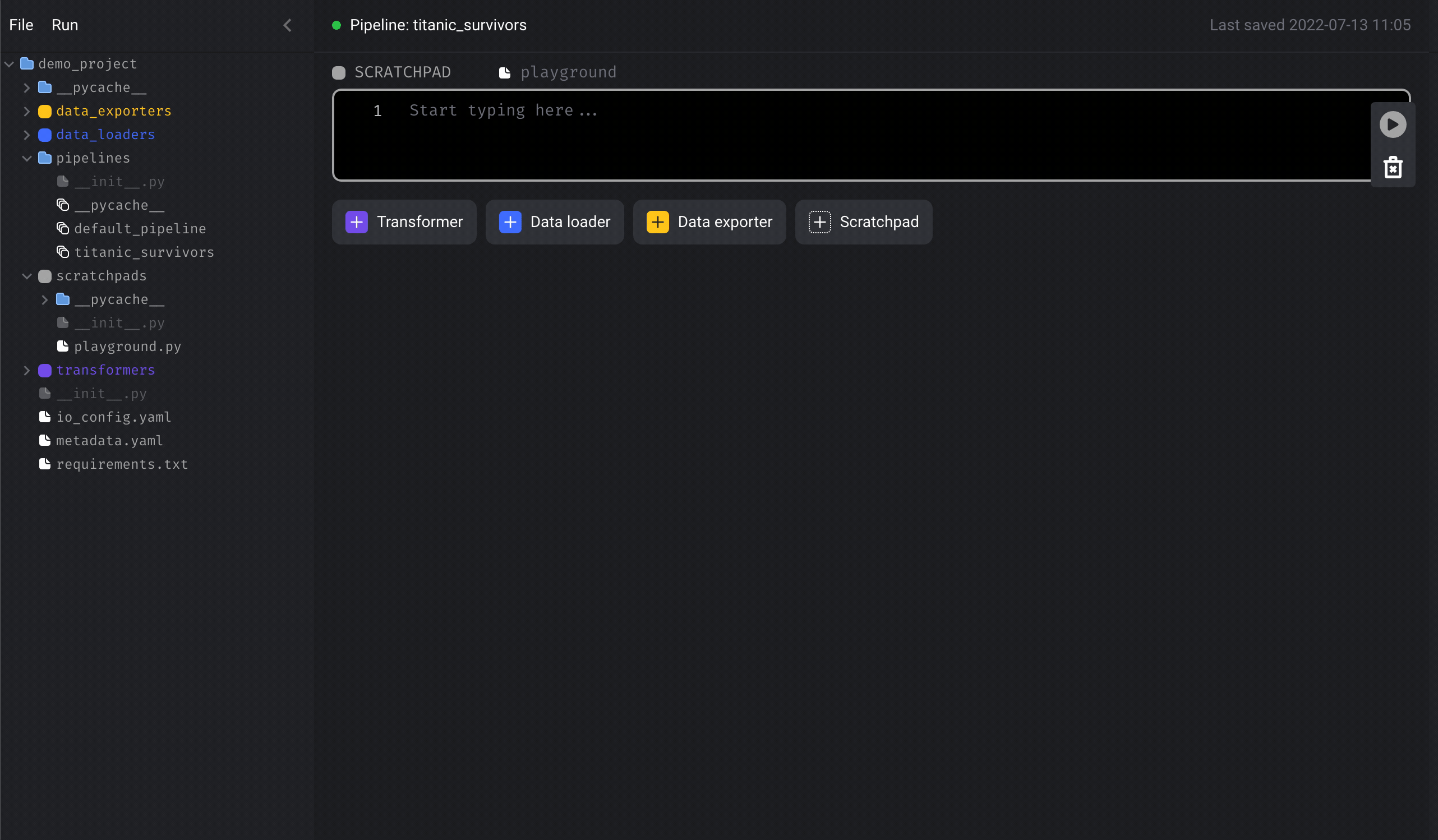Now that we’re done with the scratchpad, we can leave it there or delete it. To delete a block, click the trash can icon on the right side or use the keyboard shortcut by typing the letter D and then D again.

1. Click the `+ Data loader` button, select `Python`, then click the template called `API`.
2. Rename the block to `load dataset`.
3. In the function named `load_data_from_api`, set the `url` variable to: `https://raw.githubusercontent.com/datasciencedojo/datasets/master/titanic.csv`.
4. Run the block by clicking the play icon button or using the keyboard shortcuts `⌘ + Enter`, `Control + Enter`, or `Shift + Enter`.

After you run the block (⌘ + Enter), you can immediately see a sample of the data in the block’s output.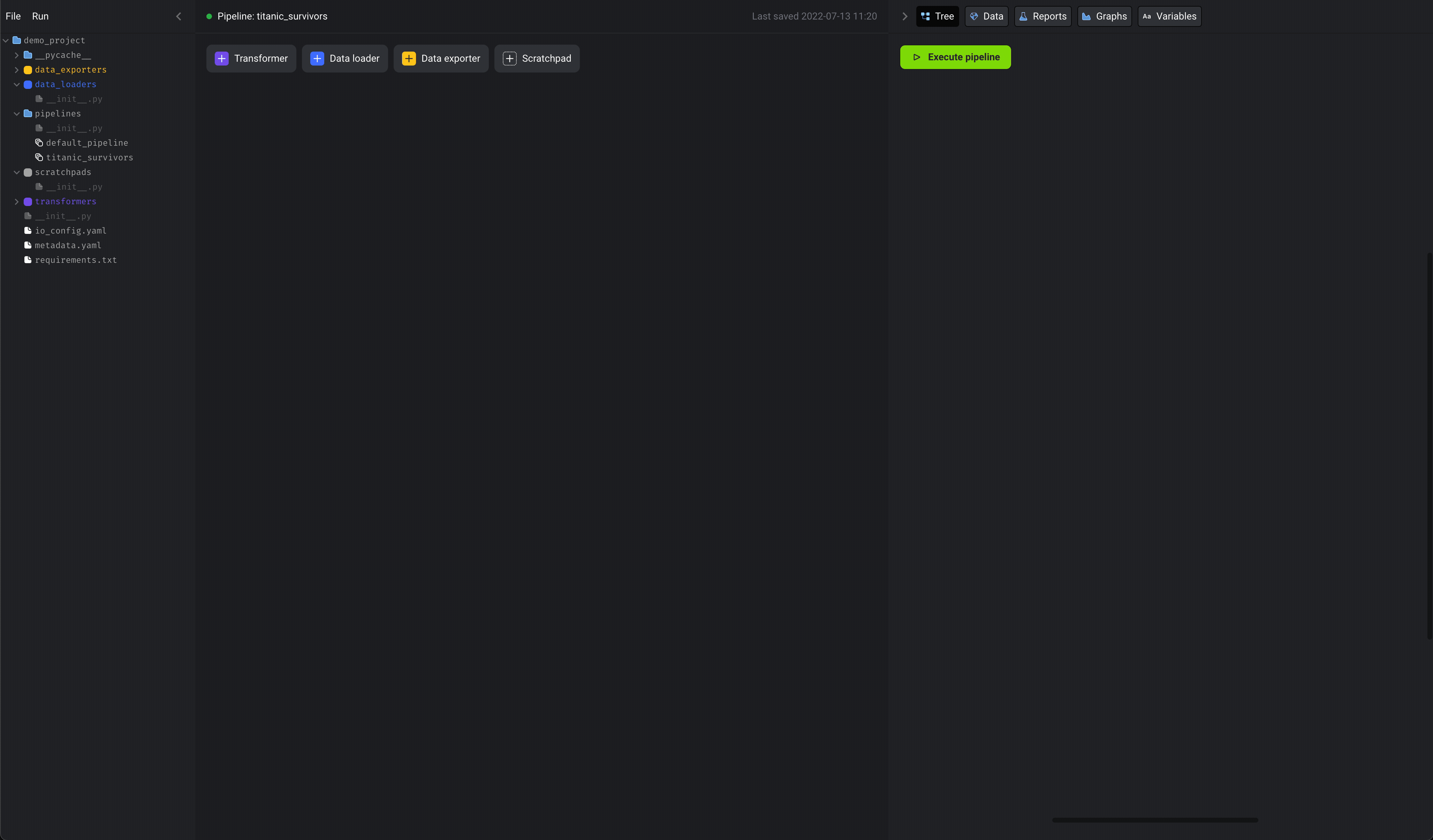Here is what the code should look like:

``````import io
import pandas as pd
import requests
from pandas import DataFrame

if 'test' not in globals():
from mage_ai.data_preparation.decorators import test

"""
"""
url = 'https://raw.githubusercontent.com/mage-ai/datasets/master/titanic_survival.csv'

response = requests.get(url)

@test
def test_output(df) -> None:
"""
Template code for testing the output of the block.
"""
assert df is not None, 'The output is undefined'
``````

## ​5. Transform data

We’re going to select numerical columns from the original dataset, then fill in missing values for those columns (aka impute).

1. Click the `+ Transformer` button, select `Python`, then click `Generic (no template)`.
2. Rename the block to `extract and impute numbers`.
3. Paste the following code in the block:
``````from pandas import DataFrame
import math

if 'transformer' not in globals():
from mage_ai.data_preparation.decorators import transformer

def select_number_columns(df: DataFrame) -> DataFrame:
return df[['Age', 'Fare', 'Parch', 'Pclass', 'SibSp', 'Survived']]

def fill_missing_values_with_median(df: DataFrame) -> DataFrame:
for col in df.columns:
values = sorted(df[col].dropna().tolist())
median_age = values[math.floor(len(values) / 2)]
df[[col]] = df[[col]].fillna(median_age)
return df

@transformer
def transform_df(df: DataFrame, *args) -> DataFrame:
return fill_missing_values_with_median(select_number_columns(df))
``````

After you run the block (⌘ + Enter), you can immediately see a sample of the data in the block’s output.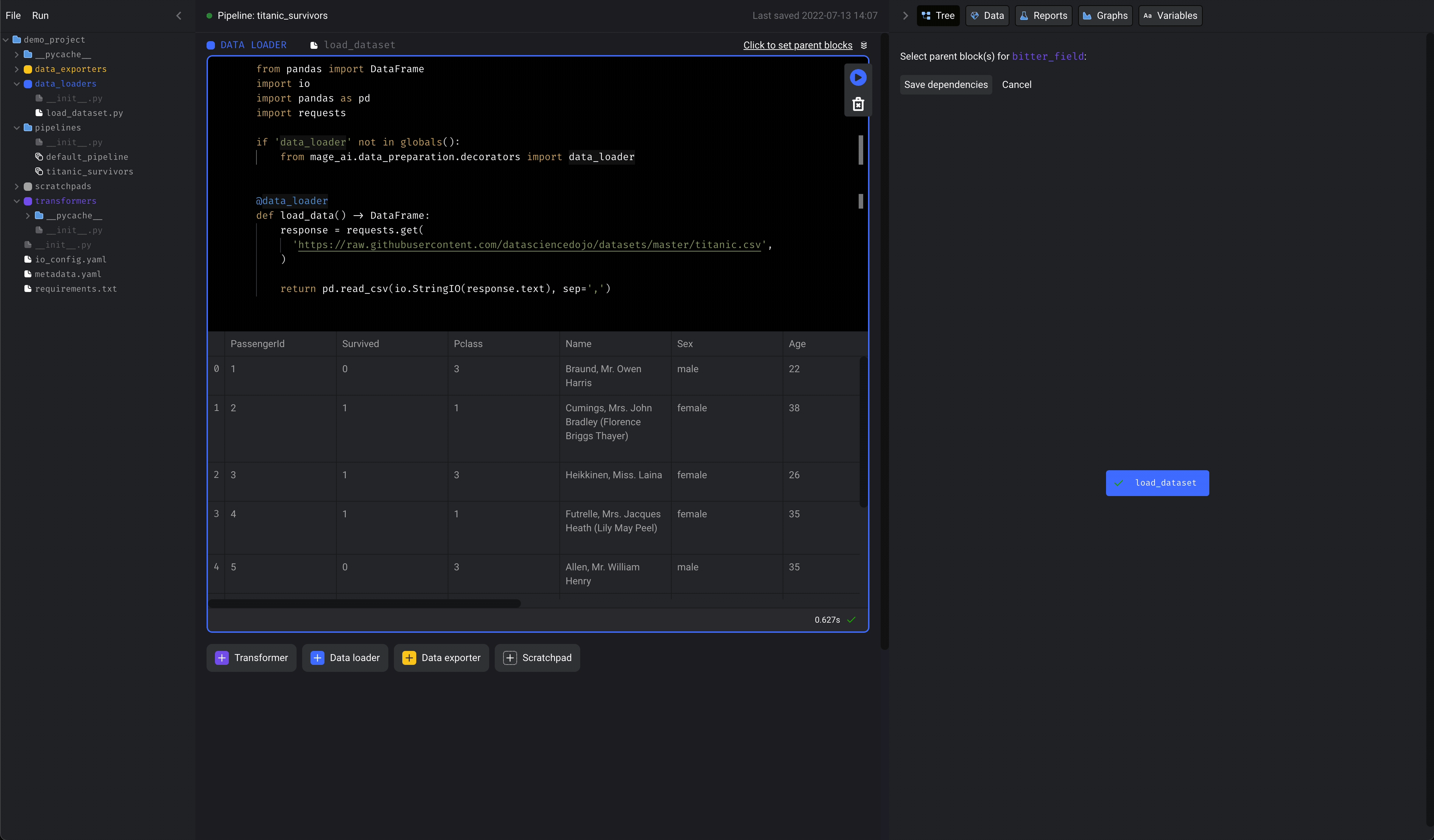## ​6. Train model

In this part, we’re going to accomplish the following:

1. Split the dataset into a training set and a test set.
2. Train logistic regression model.
3. Calculate the model’s accuracy score.
4. Save the training set, test set, and model artifact to disk.

Here are the steps to take:

1. Add a new data exporter block by clicking `+ Data exporter` button, select `Python`, then click `Generic (no template)`.
2. Rename the block to `train model`.
3. Paste the following code in the block:
``````from pandas import DataFrame
from sklearn.linear_model import LogisticRegression
from sklearn.metrics import accuracy_score
from sklearn.model_selection import train_test_split
import os
import pickle

if 'data_exporter' not in globals():
from mage_ai.data_preparation.decorators import data_exporter

LABEL_COLUMN = 'Survived'

def build_training_and_test_set(df: DataFrame) -> None:
X = df.drop(columns=[LABEL_COLUMN])
y = df[LABEL_COLUMN]

return train_test_split(X, y)

def train_model(X, y) -> None:
model = LogisticRegression()
model.fit(X, y)

return model

def score_model(model, X, y) -> None:
y_pred = model.predict(X)

return accuracy_score(y, y_pred)

@data_exporter
def export_data(df: DataFrame) -> None:
X_train, X_test, y_train, y_test = build_training_and_test_set(df)
model = train_model(X_train, y_train)

score = score_model(model, X_test, y_test)
print(f'Accuracy: {score}')

cwd = os.getcwd()
filename = f'{cwd}/finalized_model.lib'
print(f'Saving model to {filename}')
pickle.dump(model, open(filename, 'wb'))

print(f'Saving training and test set')
X_train.to_csv(f'{cwd}/X_train')
X_test.to_csv(f'{cwd}/X_test')
y_train.to_csv(f'{cwd}/y_train')
y_test.to_csv(f'{cwd}/y_test')
``````

Run the block (⌘ + Enter).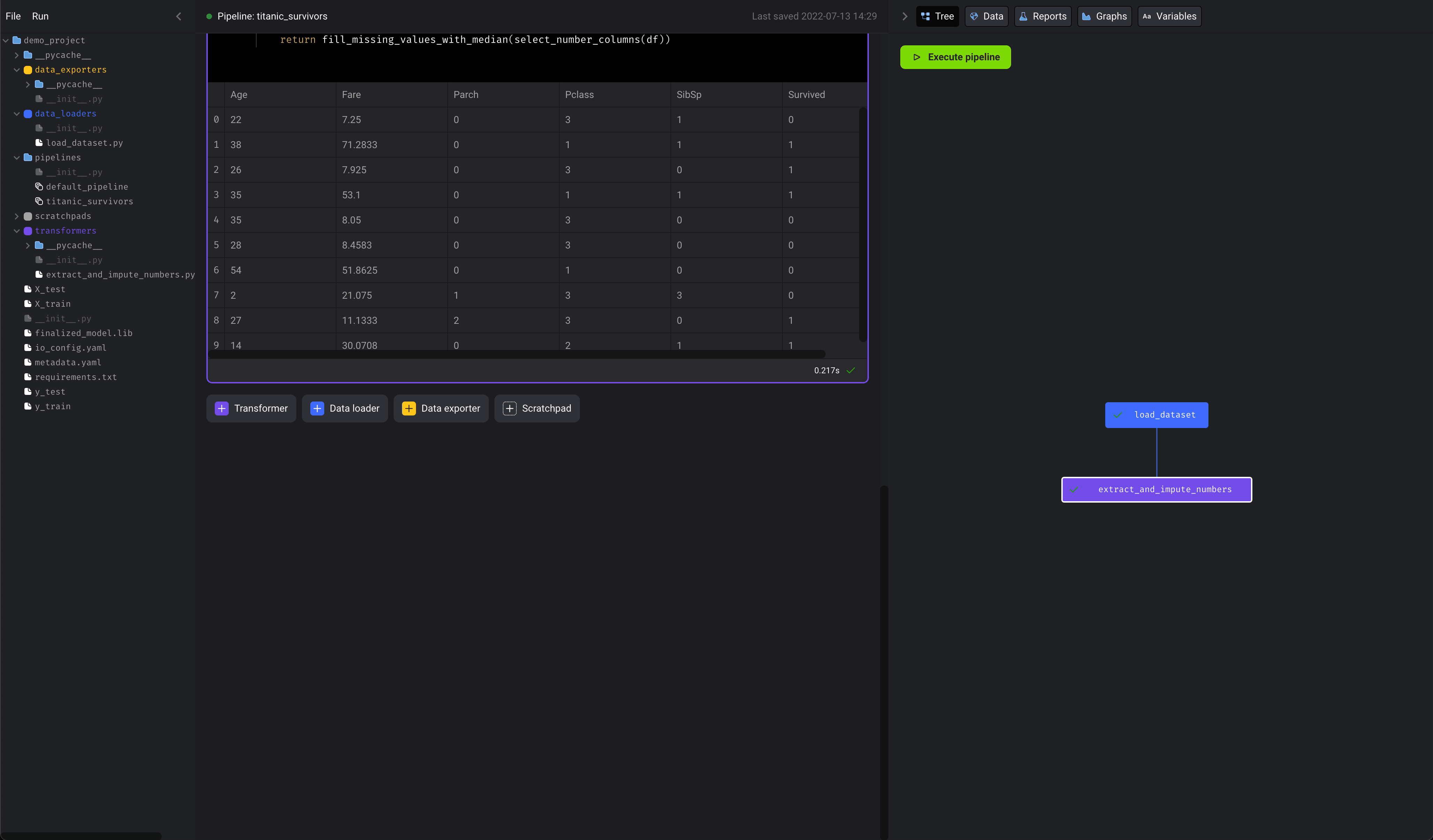## ​7. Run pipeline

We can now run the entire pipeline end-to-end. In your terminal, execute the following command:

``````./scripts/run.sh demo_project titanic_survivors
``````

You can also run the pipeline from the UI. Click on the Execute pipeline from right bottom panel.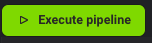Your output should look something like this:

``````Executing data_loader block: load_dataset...DONE
Executing transformer block: extract_and_impute_numbers...DONE
Executing data_exporter block: train_model...Accuracy: 0.757847533632287
Saving model to /home/src/finalized_model.lib
Saving training and test set
DONE
``````

## ​Congratulations!

You’ve successfully built an ML pipeline that consists of modular code blocks and is reproducible in any environment.

If you have more questions or ideas, please live chat with us in Slack.